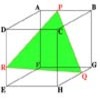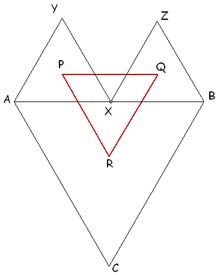#### You may also like### Cut Cube

Find the shape and symmetries of the two pieces of this cut cube.### Cushion Ball

The shortest path between any two points on a snooker table is the straight line between them but what if the ball must bounce off one wall, or 2 walls, or 3 walls?### Plane to See

P is the midpoint of an edge of a cube and Q divides another edge in the ratio 1 to 4. Find the ratio of the volumes of the two pieces of the cube cut by a plane through PQ and a vertex.

# Napoleon's Hat

##### Age 16 to 18Challenge LevelThe diagram shows three equilateral triangles $ABC, AYX$ and $XZB$. The point $X$ is a moveable point on $AB$. The points $P$, $Q$ and $R$ are the centres of the three triangles. Experiment with the dynamic diagram. What can you say about triangle $PQR$? Can you prove your conjecture?
 Created with GeoGebra